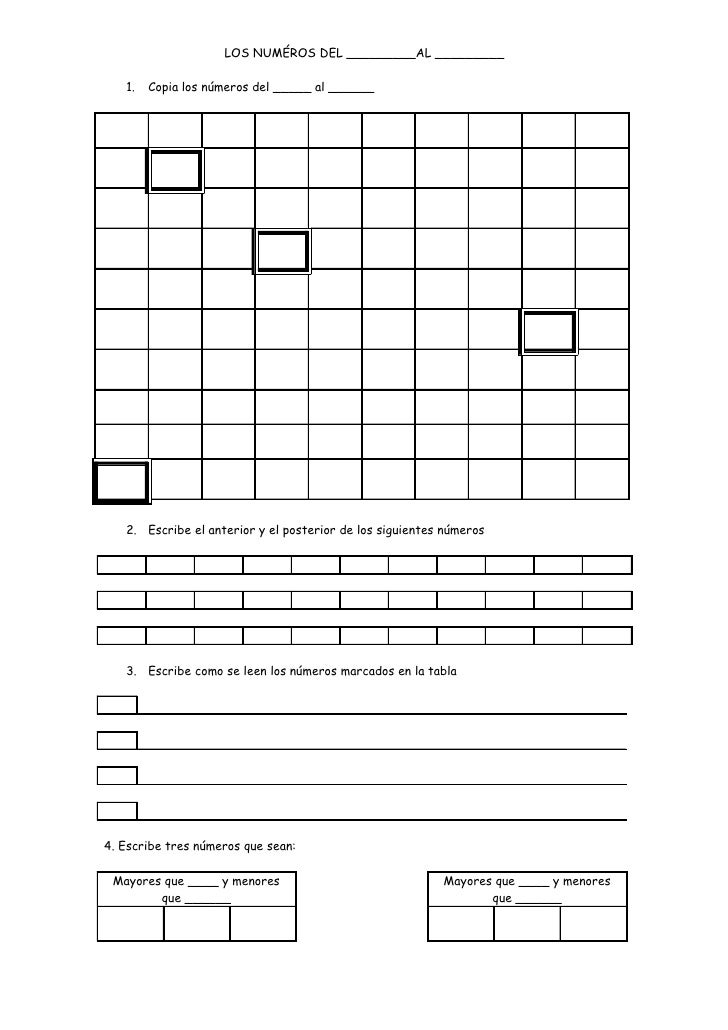Successfully reported this slideshow.
We use your LinkedIn profile and activity data to personalize ads and to show you more relevant ads. You can change your ad preferences anytime.Upcoming SlideShare
Loading in …5
×

# Ficha Numeros Y Tablas Lolo

1,019 views

Published on

Fichas para consulta e impresión del documento para mejorar el trabajo de las matemáticas en el Primer Ciclo de Primaria.

Published in: Education
• Full Name
Comment goes here.

Are you sure you want to Yes No
Your message goes here• Be the first to comment

• Be the first to like this

### Ficha Numeros Y Tablas Lolo

1. 1. LOS NUMÉROS DEL _________AL _________ 1. Copia los números del _____ al ______ 2. Escribe el anterior y el posterior de los siguientes números 3. Escribe como se leen los números marcados en la tabla 4. Escribe tres números que sean: Mayores que ____ y menores Mayores que ____ y menores que ______ que ______
2. 2. LA TABLA DEL ___ 4. Escribe la tabla del ___ como suma y pon el resultado Multiplicación Suma Resultado 5. Escribe el resultado de las siguientes multiplicaciones - ___ x ___ = ___ - ___ x ___ = ___ - ___ x ___ = ___ - ___ x ___ = ___ - ___ x ___ = ___ - ___ x ___ = ___ - ___ x ___ = ___ - ___ x ___ = ___ - ___ x ___ = ___ 6. ¿Qué multiplicación o multiplicaciones dan el siguiente resultado? - ___ = - ___ = - ___ = - ___ = - ___ = - ___ = - ___ = - ___ = - ___ =
3. 3. 7. Escribe las tablas del __________, _____ veces cada una en la siguiente cuadrícula 8. Calcula las siguientes multiplicaciones ___ ___ ___ ___ ___ ___ ___ ___ X ___ X ___ X ___ X ___ __________ __________ __________ __________ ___ ___ ___ ___ ___ ___ ___ ___ X ___ X ___ X ___ X ___ __________ __________ __________ __________ ___ ___ ___ ___ ___ ___ ___ ___
4. 4. X ___ X ___ X ___ X ___ __________ __________ __________ __________